# MATLAB做晶体结构图(固体物理).md

## 写在前面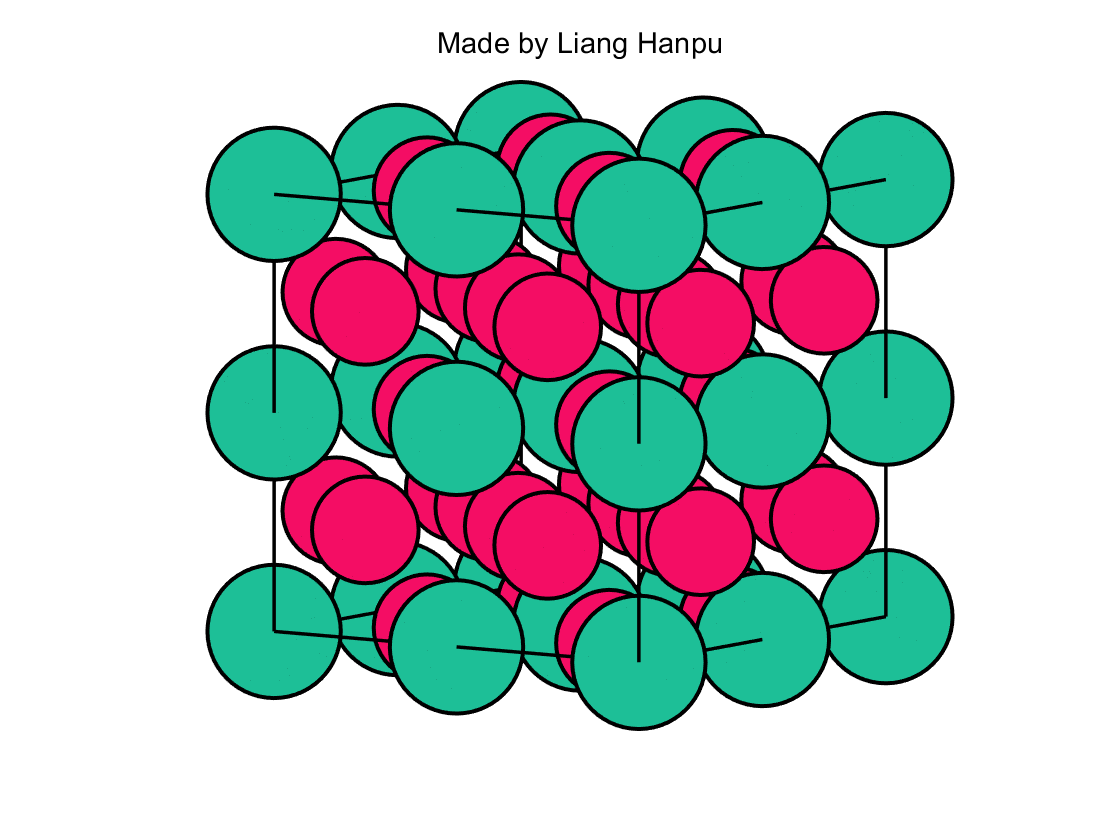## 基本的思考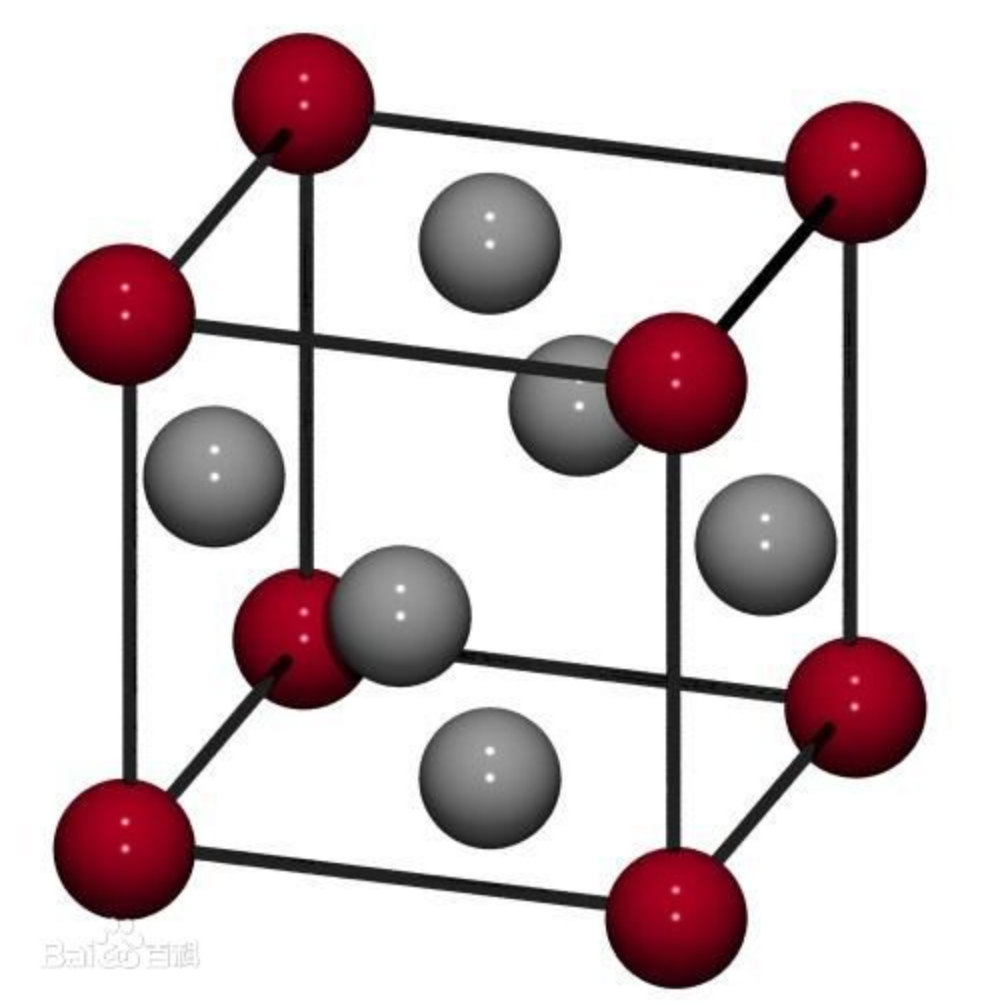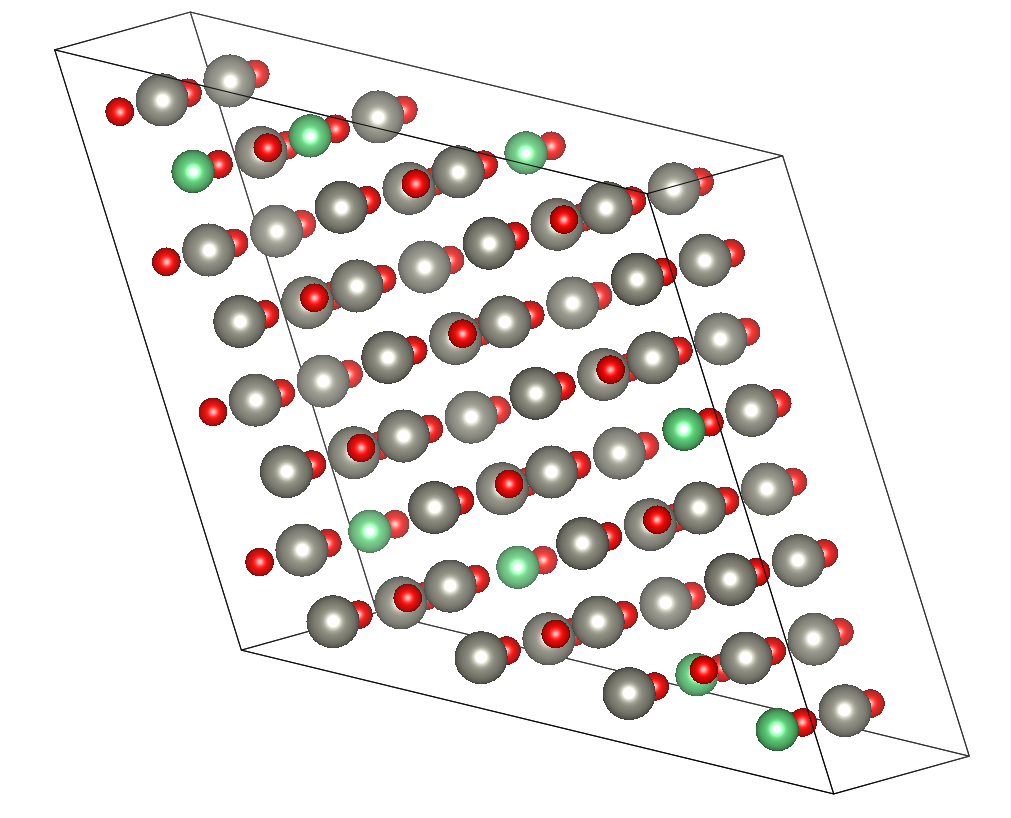• 晶胞参数：描述晶胞大小的参数。
• 超胞大小：当晶胞数量大于1个并且周期性变化时，用三个数字描述其在三个方向上的晶胞叠加数目。
• 各个原子的坐标：没有这个还怎么画出来原子啊。

## 基本参数

### 晶胞参数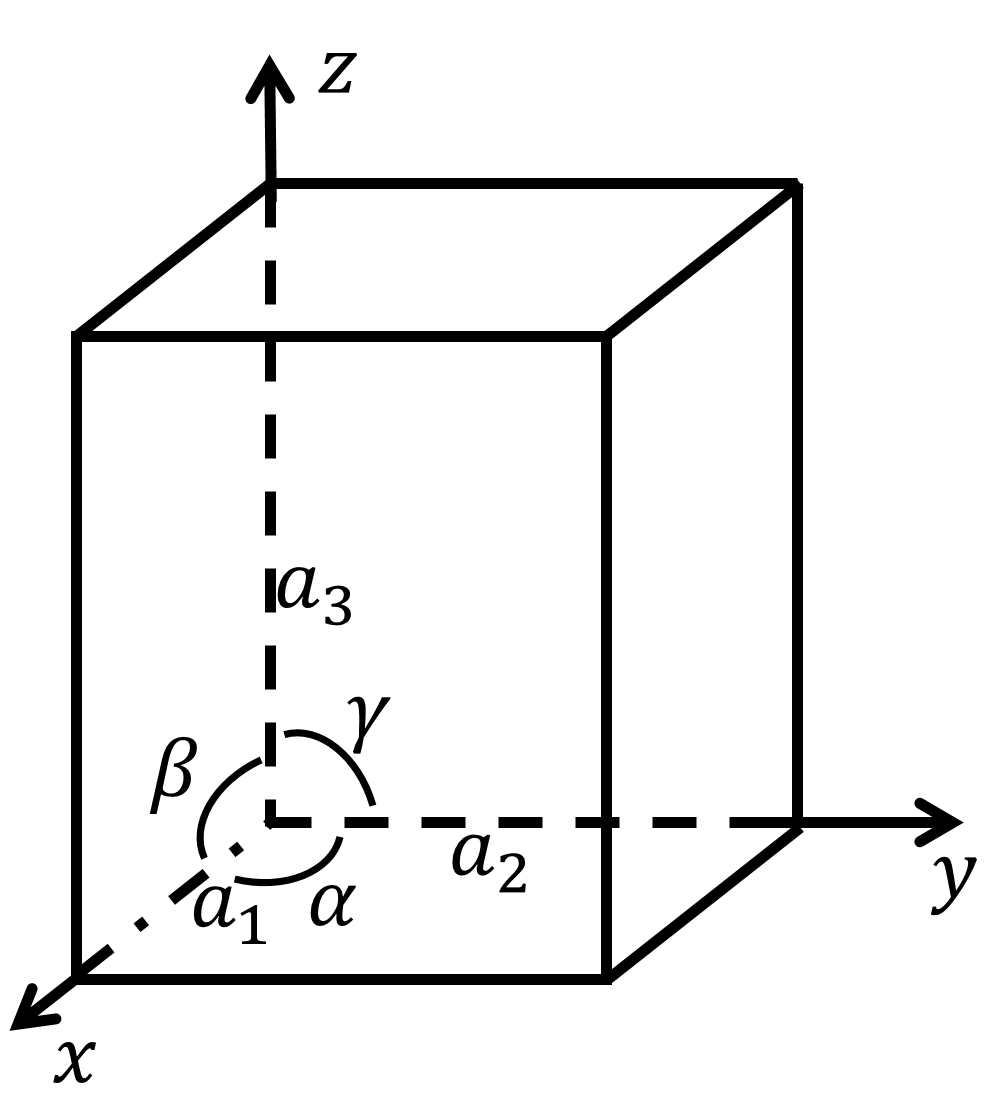### 超胞大小

**值得注意的是，这里我用的并不是$x, y, z$轴的方向！！！**因为如果$\alpha\neq 90^o$的话，那么a2方向就不和y轴平齐了，所以为了保证两个晶胞相连，就必须要沿着晶轴方向拓展。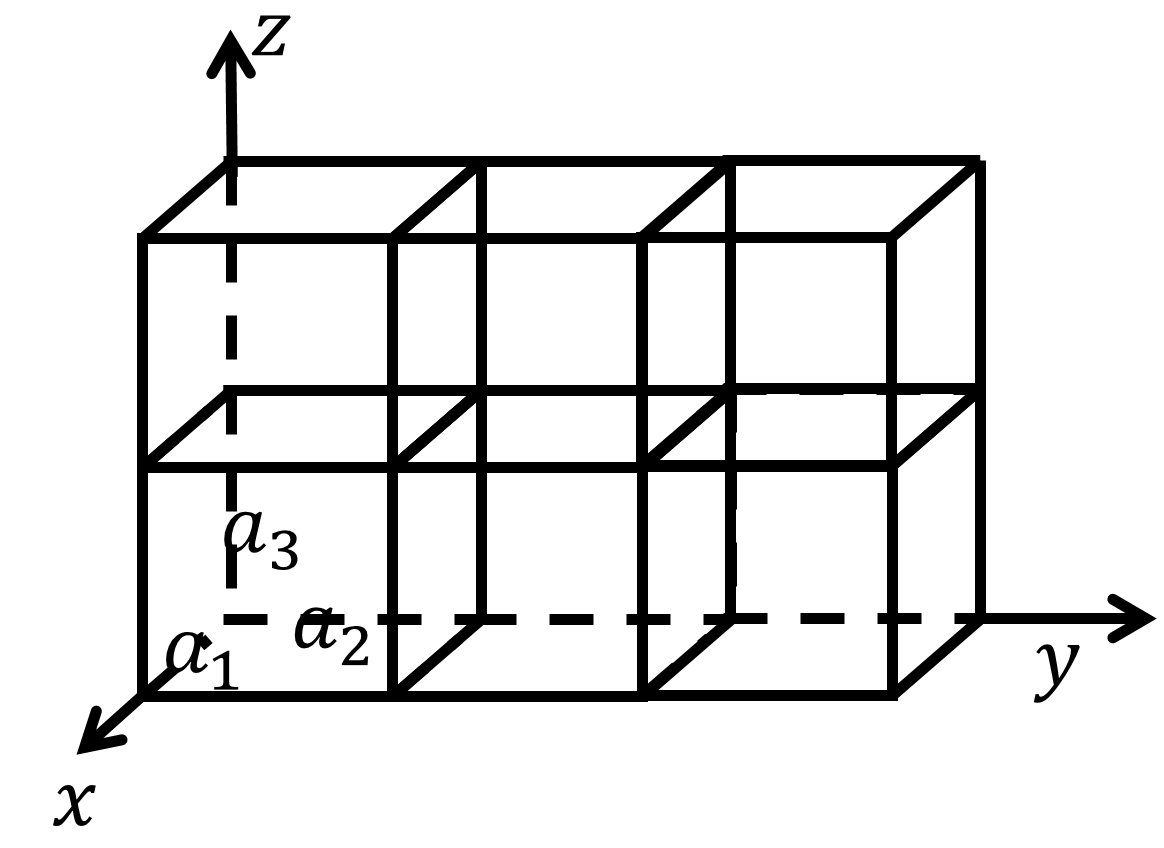## 开始写程序

### 设定参数

%% 参数设定
global cell_size a1 a2 a3 alpha beta gamma
% 晶胞参数
a = [1, 1, 1];
% 三个角度
angle = [pi/2, pi/2, pi/2];
% 超胞大小
cell_size = [2, 2, 2];
% 简单立方
position1 = [0, 0, 0; ...
1, 1, 0; ...
1, 0, 0; ...
0, 1, 0; ...
0, 0, 1; ...
1, 1, 1; ...
1, 0, 1; ...
0, 1, 1];
% 体心
position2 = [0.5, 0.5, 0.5];
% 面心
position3 = [0, 0.5, 0.5; ...
0.5, 0, 0.5; ...
0.5, 0.5, 0; ...
1, 0.5, 0.5; ...
0.5, 1, 0.5; ...
0.5, 0.5, 1];

[a1, a2, a3] = deal(a(1), a(2), a(3));
[alpha, beta, gamma] = deal(angle(1), angle(2), angle(3));


### 作图

%% 作图
figure
% 作图设置
hold on, axis equal
axis image off
view(-37.5, 30)


% 做超胞框架
plotBox();
% 做各个原子
plotAtoms(position2, [244, 13, 100]/255, 40);
plotAtoms(position1, [29, 191, 151]/255, 50);   %简单立方的一定要放在最后面


### 做超胞的框架plotBox

function plotBox()
% 做边框
global a1 a2 a3 alpha beta gamma cell_size
% 超胞的边长
[A1, A2, A3] = deal(a1*cell_size(1), a2*cell_size(2), a3*cell_size(3));
% 8个顶点
vertex = [0, 0, 0;...
A1, 0, 0;...
A2*cos(alpha), A2*sin(alpha), 0;...
A2*cos(alpha)+A1, A2*sin(alpha), 0;...
0, 0, A3;...
A1, 0, A3;...
A2*cos(alpha), A2*sin(alpha), A3;...
A2*cos(alpha)+A1, A2*sin(alpha), A3];
% 12个边
plotLine(vertex(1,:), vertex(2,:))
plotLine(vertex(1,:), vertex(3,:))
plotLine(vertex(2,:), vertex(4,:))
plotLine(vertex(3,:), vertex(4,:))
plotLine(vertex(5,:), vertex(6,:))
plotLine(vertex(5,:), vertex(7,:))
plotLine(vertex(6,:), vertex(8,:))
plotLine(vertex(7,:), vertex(8,:))
plotLine(vertex(1,:), vertex(5,:))
plotLine(vertex(2,:), vertex(6,:))
plotLine(vertex(3,:), vertex(7,:))
plotLine(vertex(4,:), vertex(8,:))
end

function plotLine(x1, x2)
% 做两个点之间的框架线
plot3([x1(1) x2(1)], [x1(2), x2(2)], [x1(3), x2(3)], 'k', 'linewidth', 1.3)
end


### 做各个原子的图

function plotAtoms(position, markercolor, markersize)
% 做各原子图像
global cell_size a1 a2 a3 alpha beta gamma
% 原始晶胞
% plot3(position(:,1), position(:,2), position(:,3), 'ok', 'linewidth', 1.5, 'markersize', 50, 'markerfacecolor', [29,191,151]/255)
% 超胞
% 遍历得到超胞所有原子
cur_point = position;
for i1 = 1:cell_size(1)
for i2=1:cell_size(2)
for i3=1:cell_size(3)
x_plus = a1*(i1-1) + a2*cos(alpha)*(i1-1);
y_plus = a2*sin(alpha)*(i2-1);
z_plus = a3*(i3-1);
cur_point = [cur_point; [position(:,1)+x_plus position(:,2)+y_plus position(:,3)+z_plus]];
end
end
end
plot3(cur_point(:,1), cur_point(:,2), cur_point(:,3), 'ok', 'linewidth', 1.5, 'markersize', markersize, 'markerfacecolor', markercolor)
% 设置坐标轴大小
[x_min, x_max, y_min, y_max, z_min, z_max] = deal(min(cur_point(:,1)), max(cur_point(:,1)), ...
min(cur_point(:,2)), max(cur_point(:,2)), ...
min(cur_point(:,3)), max(cur_point(:,3)));
x_len = (x_max - x_min)/6;
y_len = (y_max - y_min)/6;
z_len = (z_max - z_min)/6;
axis([x_min-x_len x_max+x_len y_min-y_len y_max+y_len z_min-z_len z_max+z_len])
end